# Electronics and Communication Engineering - Signals and Systems

Exercise : Signals and Systems - Section 1
11.
The z-transform of a particular signal is given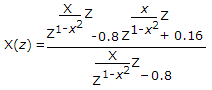where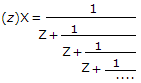The system after implementation will be
casual and stable
non-casual and stable
non-casual and unstable
casual and unstable
Explanation: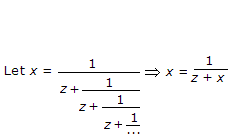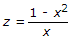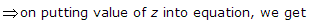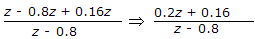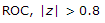Include unity circle and exterior of circle hence x(z) will be stable, causal.

12.
The system with given pole-zero diagram is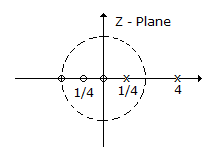casual
non-casual
both sided
not-possible
Explanation:

Because no.of zero is more than Pole, therefore when you find transfer function.

Numerator power will higher than denominator, and such transfer functions is not realizable.

13.
A pole zero pattern of a certain filter is shown in figure. This filter must be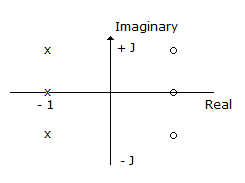LPF
BFP
HPF
APF
Explanation:

In transfer function, no of zeros = no. of poles, hence it is all pass fliter.

14.
If transfer function of a system is H(z) = 6 + z-1 + z-2 then system is
minimun phase
maximum phase
mixed phase
none
Explanation:

H(z) = 6 + z-1 - z-2, solve it by considering H(z) = 0 z = 1/3, -1/2 in H(z) only numerator.

Hence z = 1/3, - 1/2 will be zero, and if zero lies inside the unit circle, system will be of minimum phase.

15.
The period of the function cos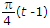is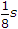8s
4s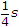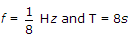.